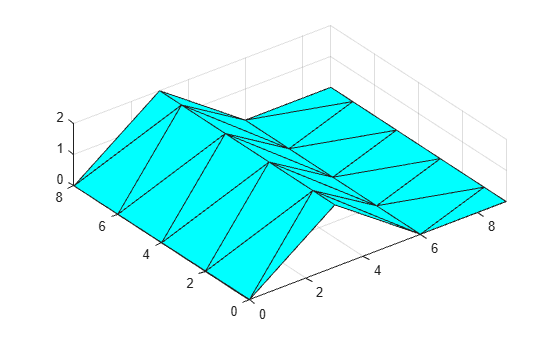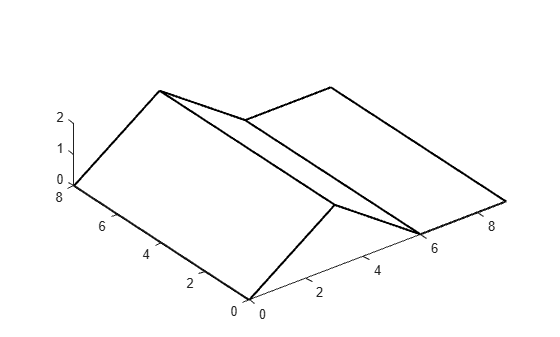# featureEdges

Sharp edges of surface triangulation

## Syntax

``F = featureEdges(TR,theta)``

## Description

example

````F = featureEdges(TR,theta)` returns the feature edges in a 2-D triangulation according to an angle `theta`. This function is typically used to extract sharp edges in a surface mesh plot.```

## Examples

collapse all

Find and plot the feature edges of a surface.

Create a 2-D Delaunay triangulation.

```x = [0 0 0 0 0 3 3 3 3 3 3 6 6 6 6 6 9 9 9 9 9 9]'; y = [0 2 4 6 8 0 1 3 5 7 8 0 2 4 6 8 0 1 3 5 7 8]'; DT = delaunayTriangulation(x,y); T = DT.ConnectivityList;```

Add elevations to the triangulation to create a surface and plot the surface.

```z = [0 0 0 0 0 2 2 2 2 2 2 0 0 0 0 0 0 0 0 0 0 0]'; trisurf(T,x,y,z,'FaceColor','cyan') axis equal```Create a new triangulation on the elevated surface, and compute and plot its feature edges using a filter angle of $\frac{\pi }{6}$.

```TR = triangulation(T,x,y,z); F = featureEdges(TR,pi/6)'; plot3(x(F),y(F),z(F),'k','LineWidth',1.5); axis equal```## Input Arguments

collapse all

Triangulation representation for 2-D triangulations only, specified as a scalar `triangulation` or `delaunayTriangulation` object.

Data Types: `triangulation` | `delaunayTriangulation`

Filter angle in radians, specified as a scalar in the range [0,π]. `featureEdges` returns adjacent triangles that have a dihedral angle that deviates from π by an angle greater than `theta`.

Data Types: `double`

collapse all

### Feature Edge

A feature edge is a triangulation edge that has any of the following attributes:

• The edge belongs to only one triangle.

• The edge is shared by more than two triangles.

• The edge is shared by a pair of triangles with angular deviation greater than the angle `theta`.

`F` is a two-column matrix whose rows correspond to a feature edge. The first element of each row is the identification number of the starting edge vertex, and the second element is the identification number of the ending edge vertex. A vertex identification is the row number of the corresponding vertex in the `Points` property.

## Version History

Introduced in R2013a